# A Really Friendly Guide To Wavelets

## Disclaimer

This tutorial is aimed at the engineer, not the mathematician. This does not mean that there will be no mathematics; it just means that there will be no proofs in the text. In my humble opinion, mathematical papers are completely unreadable because of the proofs that clutter the text. For proofs the reader is pointed to suitable references. The equations presented are there to illustrate and to clarify things, I hope. It should not be necessary to understand all the equations in order to understand the theory. However, to understand this tutorial, a mathematical background on an engineering level is required. Also some knowledge of signal processing theory might come in handy.

The information presented in this tutorial is believed to be correct. However, no responsibility whatsoever will be accepted for any damage whatsoever due to errors or misleading statements or whatsoever in this tutorial. Should there be anything incorrect, incomplete or not clear in this text, please let me know so that I can improve this tutorial.

## 1. Introduction

It is well known from Fourier theory that a signal can be expressed as the sum of a, possibly infinite, series of sines and cosines. This sum is also referred to as a Fourier expansion. However, the big disadvantage of a Fourier expansion is that it has only frequency resolution and no time resolution. This means that although we might be able to determine all the frequencies present in a signal, we do not know at what time they are present. To overcome this problem in the past decades several solutions have been developed which are more or less able to represent a signal in the time and frequency domain at the same time.

The idea behind these time-frequency joint representations is to cut the signal of interest into several parts and then analyze the parts separately. It is clear that analyzing a signal this way will give more information about the when and where of different frequency components, but it leads to a fundamental problem as well: how to cut the signal? Suppose that we want to know exactly all the frequency components present at a certain moment in time. We cut out only this very short time window using a Kronecker delta , transform it to the frequency domain and … something is very wrong.

The problem here is that cutting the signal corresponds to a convolution between the signal and the cutting window. Since convolution in the time domain is identical to multiplication in the frequency domain and since the Fourier transform of a Kronecker delta contains all possible frequencies the frequency components of the signal will be smeared out all over the frequency axis. (Please note that we are talking about a two-dimensional time-frequency transform and not a one-dimensional transform.) In fact this situation is the opposite of the standard Fourier transform since we now have time resolution but no frequency resolution whatsoever.

The underlying principle of the phenomena just described is due to Heisenberg’s uncertainty principle, which, in signal processing terms, states that it is impossible to know the exact frequency and the exact time of occurrence of this frequency in a signal. In other words, a signal can simply not be represented as a point in the time-frequency space. The uncertainty principle shows that it is very important how one cuts the signal.

The wavelet transform or wavelet analysis is probably the most recent solution to overcome the shortcomings of the Fourier transform. In wavelet analysis the use of a fully scalable modulated window solves the signal-cutting problem. The window is shifted along the signal and for every position the spectrum is calculated. Then this process is repeated many times with a slightly shorter (or longer) window for every new cycle. In the end the result will be a collection of time-frequency representations of the signal, all with different resolutions. Because of this collection of representations we can speak of a multiresolution analysis. In the case of wavelets we normally do not speak about time-frequency representations but about time-scale representations, scale being in a way the opposite of frequency, because the term frequency is reserved for the Fourier transform.

Since from literature it is not always clear what is meant by small and large scales, I will define it here as follows: the large scale is the big picture, while the small scales show the details. Thus, going from large scale to small scale is in this context equal to zooming in.

In the following sections I will present the wavelet transform and develop a scheme that will allow us to implement the wavelet transform in an efficient way on a digital computer. The transform will be so efficient that it does not even use wavelets anymore. (The careful reader might raise an eyebrow here and ask: “Surely you can’t be serious?“) 

But before we continue a disclaimer. Since wavelet theory is not a new thing anymore, it has been around now for fifteen years, say, I will not present a full and in-depth theory here. Several good textbooks on wavelet theory are available and many readable papers with a good review of wavelet theory have been published. The list of references at the end of this tutorial contains pointers to texts with more extensive wavelet theory coverage like (in no particular order) [Kai94], [Wei94], [She96], [Bur98], [Dau92], [Hub96], [Mal89], [Vet92]. I do however present some mathematical background in order to tell a coherent and clear tale (I hope).

Having this said, let’s go on to the wavelets.

## 2. The continuous wavelet transform

The wavelet analysis described in the introduction is known as the continuous wavelet transform or CWT. More formally it is written as: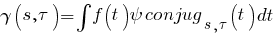(1)

where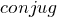denotes complex conjugation. This equation shows how a function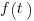is decomposed into a set of basis functions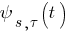called the wavelets. The variables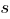and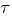, scale and translation, are the new dimensions after the wavelet transform. For completeness sake (2) gives the inverse wavelet transform. I will not expand on this since we are not going to use it: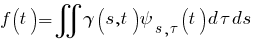(2)

The wavelets are generated from a single basic wavelet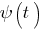, the so-called mother wavelet, by scaling and translation: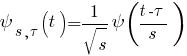(3)

In (3) s is the scale factor,is the translation factor and the factor s-1/2 is for energy normalization across the different scales.

It is important to note that in (1), (2) and (3) the wavelet basis functions are not specified. This is a difference between the wavelet transform and the Fourier transform, or other transforms. The theory of wavelet transforms deals with the general properties of the wavelets and wavelet transforms only. It defines a framework within one can design wavelets to taste and wishes.

## 3. Wavelet properties

The most important properties of wavelets are the admissibility and the regularity conditions and these are the properties which gave wavelets their name. It can be shown [She96] that square integrable functionssatisfying the admissibility condition,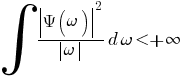(4)

can be used to first analyze and then reconstruct a signal without loss of information. In (4)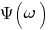stands for the Fourier transform of. The admissibility condition implies that the Fourier transform ofvanishes at the zero frequency, i.e.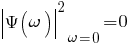(5)

This means that wavelets must have a band-pass like spectrum. This is a very important observation, which we will use later on to build an efficient wavelet transform.

A zero at the zero frequency also means that the average value of the wavelet in the time domain must be zero,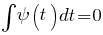(6)

and therefore it must be oscillatory. In other words,must be a wave.

As can be seen from (1) the wavelet transform of a one-dimensional function is two-dimensional; the wavelet transform of a two-dimensional function is four-dimensional. The time-bandwidth product of the wavelet transform is the square of the input signal and for most practical applications this is not a desirable property. Therefore one imposes some additional conditions on the wavelet functions in order to make the wavelet transform decrease quickly with decreasing scale s. These are the regularity conditions and they state that the wavelet function should have some smoothness and concentration in both time and frequency domains. Regularity is a quite complex concept and we will try to explain it a little using the concept of vanishing moments.

If we expand the wavelet transform (1) into the Taylor series at t = 0 until order n (let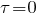for simplicity) we get [She96]: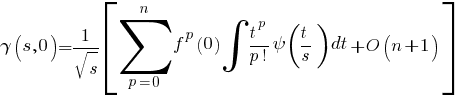(7)

Here f (p) stands for the pth derivative of f and O(n+1) means the rest of the expansion. Now, if we define the moments of the wavelet by Mp,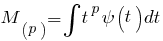(8)

then we can rewrite (7) into the finite development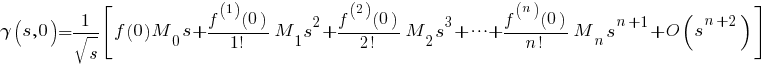(9)

From the admissibility condition we already have that the 0th moment M0 = 0 so that the first term in the right-hand side of (9) is zero. If we now manage to make the other moments up to Mn zero as well, then the wavelet transform coefficients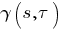will decay as fast as sn+2 for a smooth signal f(t). This is known in literature as the vanishing moments  or approximation order. If a wavelet has N vanishing moments, then the approximation order of the wavelet transform is also N. The moments do not have to be exactly zero, a small value is often good enough. In fact, experimental research suggests that the number of vanishing moments required depends heavily on the application [Cal96].

Summarizing, the admissibility condition gave us the wave, regularity and vanishing moments gave us the fast decay or the let, and put together they give us the wavelet. More about regularity  can be found for instance in [Bur98] and [Dau92].

## 4. Discrete wavelets

Now that we know what the wavelet transform is, we would like to make it practical. However, the wavelet transform as described so far still has three properties that make it difficult to use directly in the form of (1). The first is the redundancy of the CWT. In (1) the wavelet transform is calculated by continuously shifting a continuously scalable function over a signal and calculating the correlation between the two. It will be clear that these scaled functions will be nowhere near an orthogonal basis  and the obtained wavelet coefficients will therefore be highly redundant. For most practical applications we would like to remove this redundancy.

Even without the redundancy of the CWT we still have an infinite number of wavelets in the wavelet transform and we would like to see this number reduced to a more manageable count. This is the second problem we have.

The third problem is that for most functions the wavelet transforms have no analytical solutions and they can be calculated only numerically or by an optical analog computer. Fast algorithms are needed to be able to exploit the power of the wavelet transform and it is in fact the existence of these fast algorithms that have put wavelet transforms where they are today.

As mentioned before the CWT maps a one-dimensional signal to a two-dimensional time-scale joint representation that is highly redundant. The time-bandwidth product of the CWT is the square of that of the signal and for most applications, which seek a signal description with as few components as possible, this is not efficient. To overcome this problem discrete wavelets have been introduced. Discrete wavelets are not continuously scalable and translatable but can only be scaled and translated in discrete steps. This is achieved by modifying the wavelet representation (3) to create [Dau92]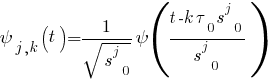(10)

Although it is called a discrete wavelet, it normally is a (piecewise) continuous function. In (10) j and k are integers and s0 > 1 is a fixed dilation step. The translation factordepends on the dilation step. The effect of making the wavelet discrete is that the time-scale space is now sampled at discrete intervals. We usually choose s0 = 2 so that the sampling of the frequency axis corresponds to dyadic sampling. This is a very natural choice for computers, the human ear and music for instance. For the translation factor we usually choose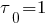so that we also have dyadic sampling of the time axis.

When discrete wavelets are used to transform a continuous signal the result will be a series of wavelet coefficients, and it is referred to as the wavelet series decomposition. An important issue in such a decomposition scheme is of course the question of reconstruction. It is all very well to sample the time-scale joint representation on a dyadic grid, but if it will not be possible to reconstruct the signal it will not be of great use. As it turns out, it is indeed possible to reconstruct a signal from its wavelet series decomposition. In [Dau92] it is proven that the necessary and sufficient condition for stable reconstruction is that the energy of the wavelet coefficients must lie between two positive bounds, i.e.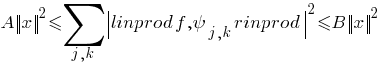(11)

where || f ||2 is the energy of f(t), A > 0, B <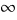and A, B are independent of f(t). When (11) is satisfied, the family of basis functions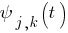withis referred to as a frame with frame bounds A and B. When A = B the frame is tight and the discrete wavelets behave exactly like an orthonormal basis. When A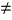B exact reconstruction is still possible at the expense of a dual frame. In a dual frame discrete wavelet transform the decomposition wavelet is different from the reconstruction wavelet.

We will now immediately forget the frames and continue with the removal of all redundancy from the wavelet transform. The last step we have to take is making the discrete wavelets orthonormal. This can be done only with discrete wavelets. The discrete wavelets can be made orthogonal to their own dilations and translations by special choices of the mother wavelet, which means: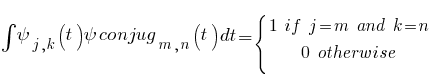(12)

An arbitrary signal can be reconstructed by summing the orthogonal wavelet basis functions, weighted by the wavelet transform coefficients [She96]:(13)

(13) shows the inverse wavelet transform for discrete wavelets, which we had not yet seen.

Orthogonality is not essential in the representation of signals. The wavelets need not be orthogonal and in some applications the redundancy can help to reduce the sensitivity to noise [She96] or improve the shift invariance of the transform [Bur98] because the discrete wavelet transform is not shift invariant, which means that the wavelet transforms of a signal and of a time-shifted version of the same signal are not simply shifted versions of each other.

## 5. A band-pass filter

With the redundancy removed, we still have two hurdles to take before we have the wavelet transform in a practical form. We continue by trying to reduce the number of wavelets needed in the wavelet transform and save the problem of the difficult analytical solutions for the end.

Even with discrete wavelets we still need an infinite number of scalings and translations to calculate the wavelet transform. The easiest way to tackle this problem is simply not to use an infinite number of discrete wavelets. Of course this poses the question of the quality of the transform. Is it possible to reduce the number of wavelets to analyze a signal and still have a useful result?

The translations of the wavelets are of course limited by the duration of the signal under investigation so that we have an upper boundary for the wavelets. This leaves us with the question of dilation: how many scales do we need to analyze our signal? How do we get a lower bound? It turns out that we can answer this question by looking at the wavelet transform in a different way.

If we look at (5) we see that the wavelet has a band-pass like spectrum. From Fourier theory we know that compression in time is equivalent to stretching the spectrum and shifting it upwards:(14)

This means that a time compression of the wavelet by a factor of two will stretch the frequency spectrum of the wavelet by a factor of two and also shift all frequency components up by a factor of two. Using this insight we can cover the finite spectrum of our signal with the spectra of dilated wavelets in the same way as that we covered our signal in the time domain with translated wavelets. To get a good coverage of the signal spectrum the stretched wavelet spectra should touch each other, as if they were standing hand in hand (see figure 2). This can be arranged by correctly designing the wavelets.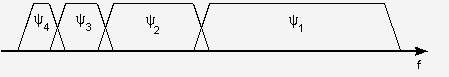Figure 2. Touching wavelet spectra resulting from scaling of the mother wavelet in the time domain.

Summarizing: if one wavelet can be seen as a band-pass filter, then a series of dilated wavelets can be seen as a band-pass filter bank. If we look at the ratio between the center frequency of a wavelet spectrum and the width of this spectrum we will see that it is the same for all wavelets. This ratio is normally referred to as the fidelity factor Q of a filter and in the case of wavelets one speaks therefore of a constant-Q filter bank.

## 6. Intermezzo: a constraint

As an intermezzo we will now take a look at an important constraint on our signal, which has sneaked in during the last section: the signal to analyze must have finite energy. When the signal has infinite energy it will be impossible to cover its frequency spectrum and its time duration with wavelets. Usually this constraint is formally stated as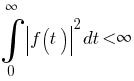(15)

and it is equivalent to saying that the L2-norm of our signal f(t) should be finite. This is where Hilbert spaces come in so we quickly end our intermezzo and only memorize that natural signals normally have finite energy.

## 7. The scaling function 

The careful reader will now ask him- or herself the question how to cover the spectrum all the way down to zero? Because every time you stretch the wavelet in the time domain with a factor of two, its bandwidth is halved. In other words, with every wavelet stretch you cover only half of the remaining spectrum, which means that you will need an infinite number of wavelets to get the job done .

The solution to this problem is simply not to try to cover the spectrum all the way down to zero with wavelet spectra, but to use a cork to plug the hole when it is small enough. This cork then is a low-pass spectrum and it belongs to the so-called scaling function. The scaling function was introduced by Mallat [Mal89]. Because of the low-pass nature of the scaling function spectrum it is sometimes referred to as the averaging filter.

If we look at the scaling function as being just a signal with a low-pass spectrum, then we can decompose it in wavelet components and express it in a way similar to (13):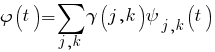(16)

Since we selected the scaling function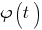in such a way that its spectrum neatly fitted in the space left open by the wavelets, the expression (16) uses an infinite number of wavelets up to a certain scale j (see figure 3). This means that if we analyze a signal using the combination of scaling function and wavelets, the scaling function by itself takes care of the spectrum otherwise covered by all the wavelets up to scale j, while the rest is done by the wavelets. In this way we have limited the number of wavelets from an infinite number to a finite number.

By introducing the scaling function we have circumvented the problem of the infinite number of wavelets and set a lower bound for the wavelets. Of course when we use a scaling function instead of wavelets we lose information. That is to say, from a signal representation point of view we do not loose any information, since it will still be possible to reconstruct the original signal, but from a wavelet-analysis point of view we discard possible valuable scale information. The width of the scaling function spectrum is therefore an important parameter in the wavelet transform design. The shorter its spectrum the more wavelet coefficients you will have and the more scale information. But, as always, there will be practical limitations on the number of wavelet coefficients you can handle. As we will see later on, in the discrete wavelet transform this problem is more or less automatically solved.

The low-pass spectrum of the scaling function allows us to state some sort of admissibility condition similar to (6):(17)

which shows that the 0th moment of the scaling function can not vanish .

Summarizing once more, if one wavelet can be seen as a band-pass filter and a scaling function is a low-pass filter, then a series of dilated wavelets together with a scaling function can be seen as a filter bank.

## 8. Subband coding

Two of the three problems mentioned in section 4 have now been resolved, but we still do not know how to calculate the wavelet transform. Therefore we will continue our journey through multiresolution land.

If we regard the wavelet transform as a filter bank, we can consider wavelet transforming a signal as passing the signal through this filter bank. The outputs of the different filter stages are the wavelet- and scaling function transform coefficients. Analyzing a signal by passing it through a filter bank is not a new idea and has been around for many years under the name subband coding. It is used for instance in computer vision applications.

The filter bank needed in subband coding can be built in several ways. One way is to build many band-pass filters to split the spectrum into frequency bands. The advantage is that the width of every band can be chosen freely, in such a way that the spectrum of the signal to analyze is covered in the places where it might be interesting. The disadvantage is that we will have to design every filter separately and this can be a time consuming process. Another way is to split the signal spectrum in two (equal) parts, a low-pass and a high-pass part. The high-pass part contains the smallest details we are interested in and we could stop here. We now have two bands. However, the low-pass part still contains some details and therefore we can split it again. And again and again, until we are satisfied with the number of bands we have created. In this way we have created an iterated filter bank. Usually the number of bands is limited by for instance the amount of data or computation power available. The process of splitting the spectrum is graphically displayed in figure 4. The advantage of this scheme is that we have to design only two filters; the disadvantage is that the signal spectrum coverage is fixed.

Looking at figure 4 we see that what we are left with after the repeated spectrum splitting is a series of band-pass bands with doubling bandwidth and one low-pass band. (Although in theory the first split gave us a high-pass band and a low-pass band, in reality the high-pass band is a band-pass band due to the limited bandwidth of the signal.) In other words, we can perform the same subband analysis by feeding the signal into a bank of band-pass filters of which each filter has a bandwidth twice as wide as his left neighbor (the frequency axis runs to the right here) and a low-pass filter. At the beginning of this section we stated that this is the same as applying a wavelet transform to the signal. The wavelets give us the band-pass bands with doubling bandwidth and the scaling function provides us with the low-pass band. From this we can conclude that a wavelet transform is the same thing as a subband coding scheme using a constant-Q filter bank  [Mal89]. In general we will refer to this kind of analysis as a multiresolution analysis.

Summarizing, if we implement the wavelet transform as an iterated filter bank, we do not have to specify the wavelets explicitly! This sure is a remarkable result .

## 9. The discrete wavelet transform

In many practical applications the signal of interest is sampled. In order to use the results we have achieved so far with a discrete signal we have to make our wavelet transform discrete too. Remember that our discrete wavelets are not time-discrete, only the translation- and the scale step are discrete. Simply implementing the wavelet filter bank as a digital filter bank intuitively seems to do the job. But intuitively is not good enough, we have to be sure.

In (16) we stated that the scaling function could be expressed in wavelets from minus infinity up to a certain scale j. If we add a wavelet spectrum to the scaling function spectrum we will get a new scaling function, with a spectrum twice as wide as the first. The effect of this addition is that we can express the first scaling function in terms of the second, because all the information we need to do this is contained in the second scaling function. We can express this formally in the so-called multiresolution formulation [Bur98] or two-scale relation [She96]: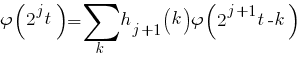(18)

The two-scale relation states that the scaling function at a certain scale can be expressed in terms of translated scaling functions at the next smaller scale. Do not get confused here: smaller scale means more detail.

The first scaling function replaced a set of wavelets and therefore we can also express the wavelets in this set in terms of translated scaling functions at the next scale. More specifically we can write for the wavelet at level j: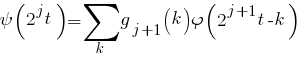(19)

which is the two-scale relation between the scaling function and the wavelet.

Since our signal f(t) could be expressed in terms of dilated and translated wavelets up to a scale j-1, this leads to the result that f(t) can also be expressed in terms of dilated and translated scaling functions at a scale j: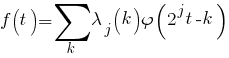(20)

To be consistent in our jargon we should in this case speak of discrete scaling functions since only discrete dilations and translations are allowed.

If in this equation we step up a scale to j-1 (!), we have to add wavelets in order to keep the same level of detail. We can then express the signal f(t) as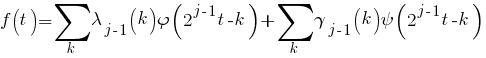(21)

If the scaling function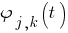and the waveletsare orthonormal or a tight frame, then the coefficients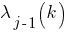and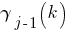are found by taking the inner products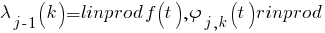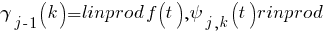(22)

If we now replaceandin the inner products by suitably scaled and translated versions  of (18) and (19) and manipulate a bit, keeping in mind that the inner product can also be written as an integration, we arrive at the important result [Bur98]:(23)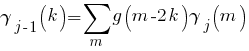(24)

These two equations state that the wavelet- and scaling function coefficients on a certain scale can be found by calculating a weighted sum of the scaling function coefficients from the previous scale. Now recall from the section on the scaling function that the scaling function coefficients came from a low-pass filter and recall from the section on subband coding how we iterated a filter bank by repeatedly splitting the low-pass spectrum into a low-pass and a high-pass part. The filter bank iteration started with the signal spectrum, so if we imagine that the signal spectrum is the output of a low-pass filter at the previous (imaginary) scale, then we can regard our sampled signal as the scaling function coefficients from the previous (imaginary) scale. In other words, our sampled signal f(k) is simply equal to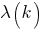at the largest scale!

But there is more. As we know from signal processing theory a discrete weighted sum like the ones in (23) and (24) is the same as a digital filter and since we know that the coefficients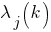come from the low-pass part of the splitted signal spectrum, the weighting factors h(k) in (23) must form a low-pass filter. And since we know that the coefficientscome from the high-pass part of the splitted signal spectrum, the weighting factors g(k) in (24) must form a high-pass filter. This means that (23) and (24) together form one stage of an iterated digital filter bank and therefore from now on we will refer to the coefficients h(k) as the scaling filter and the coefficients g(k) as the wavelet filter.

By now we have made certain that implementing the wavelet transform as an iterated digital filter bank is possible and from now on we can speak of the discrete wavelet transform or DWT. Our intuition turned out to be correct. Because of this we are rewarded with a useful bonus property of (23) and (24), the subsampling property. If we take one last look at these two equations we see that the scaling and wavelet filters have a step-size of 2 in the variable k. The effect of this is that only every otheris used in the convolution, with the result that the output data rate is equal to the input data rate. Although this is not a new discovery, it has always been exploited in subband coding schemes, it is kind of nice to see it pop up here as part of the deal.

The subsampling property also solves our problem which had come up at the end of the section on the scaling function: how to choose the width of the scaling function spectrum. Because every time we iterate the filter bank, the number of samples for the next stage is halved so that in the end we are left with just one sample (in the extreme case). It will be clear that this is where the iteration definitely has to stop and this determines the width of the spectrum of the scaling function. Normally the iteration will stop at the point where the number of samples has become smaller than the length of the scaling filter or the wavelet filter, whichever is the longest, so the length of the longest filter determines the width of the spectrum of the scaling function.

## 10. Coda

By now we have managed to reduce the highly redundant continuous wavelet transform as formulated in (1) with its infinite number of unspecified wavelets to a finite stage iterated digital filter bank which can be directly implemented on a digital computer. The redundancy has been removed by using discrete wavelets and a scaling function solved the problem of the infinite number of wavelets needed in the wavelet transform. The filter bank has solved the problem of the non-existence of analytical solutions as mentioned in the section on discrete wavelets. Finally, we have built a digitally implementable version of (1) without specifying any wavelet, just as in (1). The wavelet transform has become very practical indeed.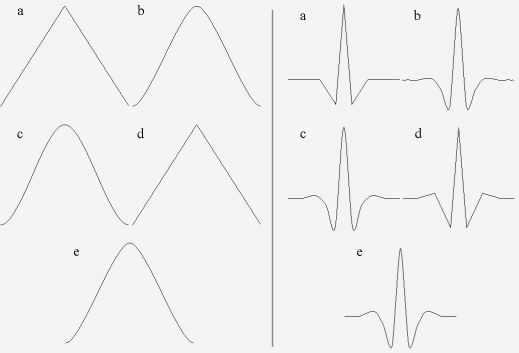Figure 6. Some scaling functions (left) and wavelets (rigth) from the biorthogonal Deslauriers-Dubuc family (like humans wavelets live in families, but there are also species that live in packs). From left to right: (2,2), (4,2), (6,2), (2,4) and (4,4). The first number is the number of vanishing moments of the analyzing wavelet (the wavelet that decomposes a signal) and the second number is the number of vanishing moments of the synthesizing wavelet (the wavelet that reconstructs the signal). Note that with increasing number of vanishing moments (wavelets a, b and c) the wavelet becomes smoother or more regular. The same is true for the scaling function. Note also that the shape of these wavelets is not the rule. Although there are many wavelets that look like this, there are also many wavelets that look completely different. The wavelets shown here are just easy to create.

## 11. Notes

 This in contrast to [Kai94].

 A Kronecker delta is defined as f (t) = 1 at t=0 and f (t) = 0 for all other t.

I am serious, and don’t call me Shirley.” Leslie Nielsen as Dr. Rumack in the film Airplane! (1980).

 There exist functions of which all moments vanish. An example is the function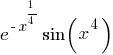for x≥0 [Kör96]

 The term regularity seems to stem from the definition that a filter is called K-regular if its z-transform has K zeroes at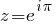. In wavelet theory this applies to the scaling filter (which has not been mentioned yet) and it is possible only if all wavelet moments up to K-1 vanish [Bur98]. The scaling filter is formed by the coefficients h(k) in (17).

 The CWT behaves just like an orthogonal transform in the sense that the inverse wavelet transform permits us to reconstruct the signal by an integration of all the projections of the signal onto the wavelet basis. This is called quasi-orthogonality [She96].

 Note that this introduction of the scaling function differs from the usual introduction through multiresolution analysis. I hope that by doing it this way it will keep the story gripping.

 When you want to go from A to B you first have to travel half the distance. But before you reach this half-way point you have to travel half of half the distance. But before you reach this quarter-way point you have to travel half of half of half the distance, etc.. In other words, you will never arrive in B because you have to travel to an infinite number of half-way points. This is the famous dichotomy paradox by Zeno of Elea (approx. 490-430 BC).

 If the degrees of freedom in a wavelet transform design are not only used on creating vanishing moments for the wavelet, but are equally distributed among the scaling function and the wavelet to create vanishing moments for both functions, we speak of Coiflets. Coiflets are more symmetric than the wavelet transforms known before their introduction (1989). They are named after their inventor Coifman [Tia96].

 Subband coding is not restricted to constant-Q filter banks.

 Since it is possible to implement a wavelet transform without explicitly implementing wavelets, it might be a good idea not to use the term wavelet transform at all and just call it subband coding. But then again, the Fourier transform can be implemented without explicitly implementing Fouriers, so why bother?

 To scale and translate suitably, replace 2jt in (18) and (19) by 2jt-k.

## 12. References

Books and papers

[Bur98] Burrus, C. S. and R. A. Gopinath, H. Guo. INTRODUCTION TO WAVELETS AND WAVELET TRANSFORMS, A PRIMER. Upper Saddle River, NJ (USA): Prentice Hall, 1998.

[Cal96] Calderbank, A. R. and I. Daubechies, W. Sweldens, B.-L. Yeo WAVELET TRANSFORMS THAT MAP INTEGERS TO INTEGERS. Proceedings of the IEEE Conference on Image Processing. Preprint, 1996. IEEE Press, 1997.

[Dau92] Daubechies, I. TEN LECTURES ON WAVELETS. 2nd ed. Philadelphia: SIAM, 1992. CBMS-NSF regional conference series in applied mathematics 61.

[Hub96] Hubbard, B. Burke THE WORLD ACCORDING TO WAVELETS. Wellesey, MA (USA): A K Peters, 1996.

[Kai94] Kaiser, G. A FRIENDLY GUIDE TO WAVELETS. Boston: Birkhäuser, 1994.

[Kör96] Körner, T. W. FOURIER ANALYSIS. Cambridge, UK: Cambridge University Press, 1996.

[Mal89] Mallat, S. G. A THEORY FOR MULTIRESOLUTION SIGNAL DECOMPOSITION: THE WAVELET REPRESENTATION. IEEE Transactions on Pattern Analysis and Machine Intelligence, Vol. 11, No. 7 (1989), p. 674-693.

[She96] Sheng, Y. WAVELET TRANSFORM. In: The transforms and applications handbook. Ed. by A. D. Poularikas. P. 747-827. Boca Raton, Fl (USA): CRC Press, 1996. The Electrical Engineering Handbook Series.

[Vet92] Vetterli M. and C. Herley. WAVELETS AND FILTER BANKS: THEORY AND DESIGN. IEEE Transactions on Signal Processing, Vol. 40, No. 9 (1992), p. 2207-2232.

[Wei94] Weiss, L. G. WAVELETS AND WIDEBAND CORRELATION PROCESSING. IEEE Signal Processing Magazine, January (1994), p. 13-32.

Internet resources

Besides traditional references (i.e. on paper) there are many Internet resources that deal with wavelets. Here I list a few that have proved to be useful. With these links probably every other wavelet related site can be found. Keep in mind however that this list was verified for the last time in October 2010.

The Wavelet Digest, a monthly electronic magazine currently edited by Wim Sweldens, is a platform for people working with wavelets. It contains announcements of conferences, abstracts of publications and preprints and questions and answers of readers. It can be found at and it is the site for wavelets.

Rice University, the home of Burrus [Bur98] et al, keeps a list of publications and makes available the Rice Wavelet Toolbox for MatLab.

The Katholieke Universiteit of Leuven, Belgium, is active on the net with wavelets, publications and the toolbox WAILI.

Amara Graps maintains a long list of links, publications and tools besides explaining wavelet theory in a nutshell.

There used to be a real lifting page, dedicated to Liftpack, a lifting toolbox. Unfortunately, it is no longer available but recently I found a copy on an old hard disk. Download it here.